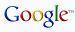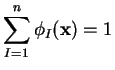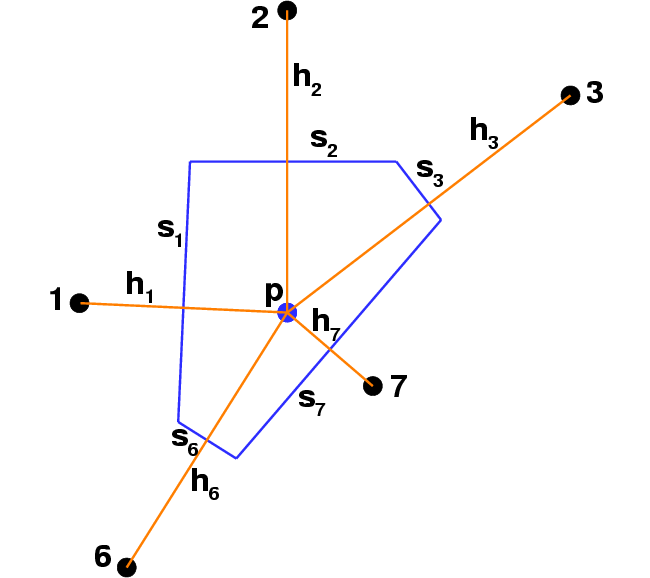Introduction
Home
CV
Publications
Directions

Research
NEM X-FEM MAXENT
POLYFEM
QUANTUM

Teaching
ECI212A ENG104

Programming
C++ C++ FAQ
STL Python
PS CTAN CPAN

Links
E-Journals
Linux RPMs
Websters
Dilbert

Societies
USACM IUTAM

Sports
Running [Marathons]
Chess TT NBATHE NATURAL ELEMENT METHOD IN SOLID MECHANICS#### Natural neighbor basis function

Introduction

Natural neighbor interpolation is a multivariate scattered data interpolation method, which has been used in geophysical modeling and GIS, as trial and test approximations in a Galerkin method (natural element method), surface parametrization, animation, computer graphics, etc. Natural neighbor (n-n) coordinates were introduced by Sibson (1980) as a means for data interpolation and smoothing. These interpolants are constructed on the basis of the underlying Voronoi tessellation which partitions a given set of nodes. The natural neighbor interpolant has the following important properties:

• Partition of unity (PU)• Non-negativity and convexity of trial function due to PU property• Cardinal property and interpolation• Linear completeness (can exactly reproduce linear displacement fields)• Compact support, and hence a local interpolation scheme is realized

• Smooth everywhere, except at the nodes where they are C0()The Sibson basis function is defined as (p is a point with coordinate x):The application of natural neighbor coordinates to the numerical solution of partial differential equations (PDEs) was carried out by Traversoni (1994) and Braun and Sambridge (1995). The latter researchers coined the name Natural Element Method (NEM) to refer to its numerical implementation. In NEM, the trial and test functions are constructed using natural neighbor coordinates. A standard Galerkin procedure is used to obtain the discrete system: Kd = f, which is solved for the unknown displacement vector d. In one-dimension, NEM is identical to linear finite elements. My thesis-work focused on the application of NEM to elliptic BVPs in solid mechanics (Sukumar (1998), Sukumar et al. (1998)).

Smooth (C1) Natural Neighbor Interpolant

Farin (1990) has constructed a C1 interpolant by embedding natural neighbor coordinates in the Bernstein-Bezier surface representation of a cubic simplex. The interpolant has quadratic completeness in, and reduces to a cubic polynomial between adjacent nodes on the boundary. I have proposed a computational methodology for its numerical implementation (NEM) for partial differential equations. The approach involves the transformation of the original Bernstein basis functionsthat appear in Farin's interpolant to new basis functions, such that the basis functions,, andfor node I are directly associated with the three nodal degrees of freedom,, and, respectively. The basis functions interpolate to nodal function and nodal gradient values, which renders the interpolant amenable to application in a Galerkin scheme for the solution of fourth-order elliptic PDEs. In one-dimension, the C1 NEM interpolant is identical to cubic Hermite finite elements. The C1 conforming implementation of NEM has been applied to the biharmonic equation with Dirichlet boundary conditions (Sukumar and Moran, 1998).#### Smooth (C1) n-n basis functions [and]

Laplace Interpolation

#### Introduction

Christ et al. (1982) were the first to propose the so-called boundary-to-distance weight measureduring the course of their studies on random lattices. In the late 1990s, the interpolant was re-discovered independently by Belikov et al. (1997) (non-Sibsonian) and Hiyoshi and Sugihara (1999) (Laplace). The Laplace interpolation method is also a natural neighbor-based interpolation scheme. An overview of the Sibson and Laplace interpolants appears in Sukumar (2003). These two interpolants share many common properties, such as partition of unity, linear completeness, compact support, etc.

We present the Laplace interpolant for the 2D case. Refer to the nodal set and Voronoi diagram shown earlier, where the point p with coordinate x has five natural neighbors (p does not lie within the three circumcircles shown in green). The Voronoi cell of the point p and its neighbors are illustrated in the figure below.#### Voronoi cell of p and the Laplace interpolant

The quantity sI(x) is the Lebesgue measure (length in R2) of the Voronoi edge associated with node I, and hI(x) is the distance between p and node I. The Laplace basis function is defined as:In a general d-dimensional setting, the computational complexity of the Laplace basis function depends on the ratio of a Lebesgue measure of Rd divided by a linear dimension. An immediate comparison with the Sibson basis function reveals that the computational effort in Rd for the Sibson interpolant is co-dimensional, whereas for the Laplace interpolant it is one order less. I collaborated with Dr. Andrei Yu Semenov on the use of the Laplace interpolant within a Galerkin framework for applications in 2D linear elasticity (Sukumar et al. (2001)).

The advent of meshless and particle methods has provided impetus to explore collocation and finite-difference methods that are based on lattice sites (nodes) alone. This is as opposed to continuum computations (e.g., FEM) in which the connectivity between the nodes define the elements that are used in the numerical integration. To explore the approximation of the discrete diffusion operator on unstructured grids (irregular lattices), in Sukumar (2003) a finite difference scheme on Voronoi grids was studied. On regular grids, the difference (collocation) scheme reduces to well-known finite difference stencils; consistency (theoretical) and convergence (through numerical examples) on non-uniform grids in 2D are also investigated.

Reports and Publications

ThesisPh.D. Thesis: The Natural Element Method in Solid Mechanics, Evanston, IL, U.S.A., June 1998.

Presentations

Fortran Code

Natural Neighbor Interpolation and NEM

Computational Geometry

You are visitor number to this page since June 06, 2002.

© Copyright 1998-2017, N. Sukumar. All rights reserved.